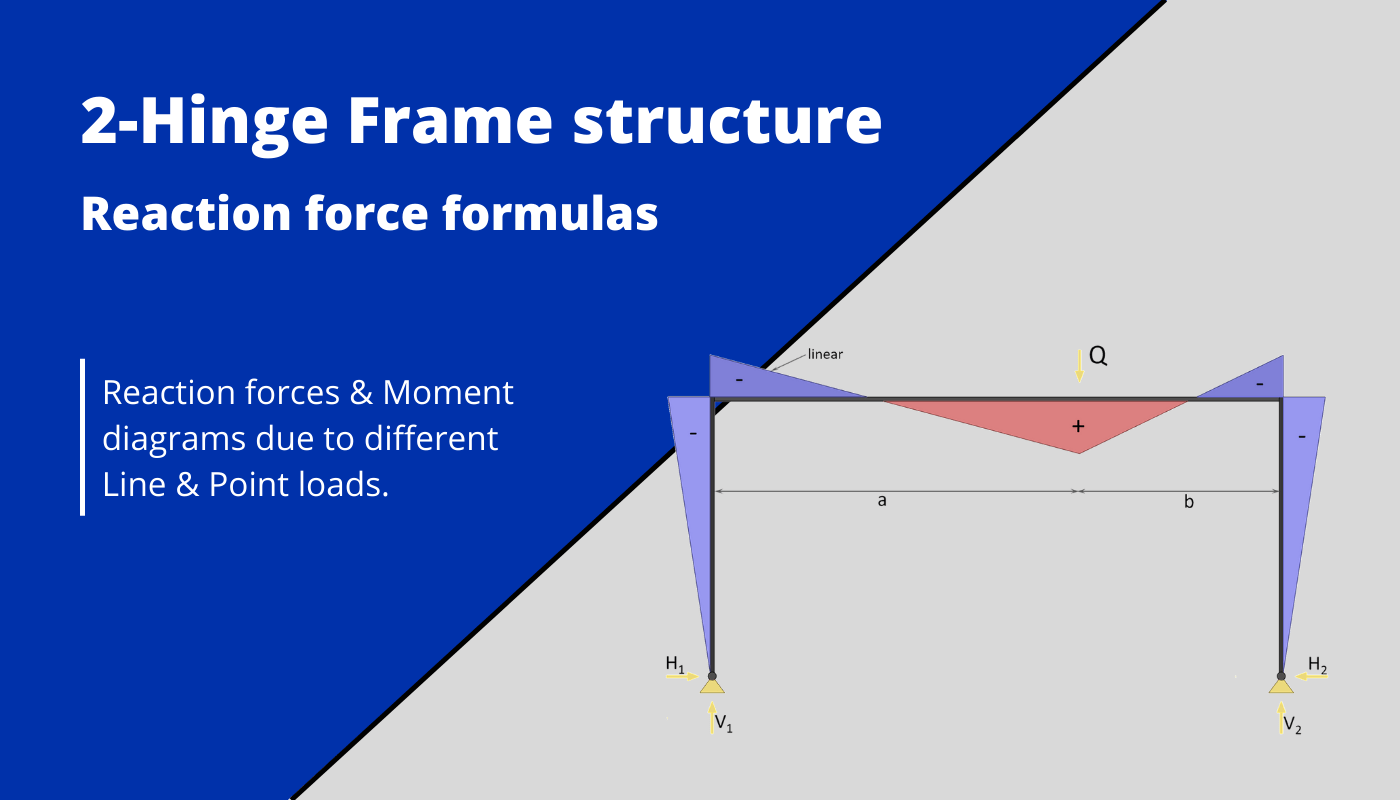# Two-hinge frame structure: Reaction force formulas – Different loadsLast updated: November 8th, 2022

Calculating bending moments, shear and reaction forces in structural elements – in this case frames with two hinged supports – for different loading scenarios, is probably one of the things in structural engineering that we do throughout our studies and also careers later on.

While it’s very important to know how to derive and calculate reaction and internal forces, the further we get in our studies, the more we can use frame moment and shear formulas.

In earlier articles, we already covered moment and shear force formulas and diagrams for:

• Simply supported beams
• Cantilever beams
• 2 span continuous beams
• 3 span continuous beams
• 4 span continuous beams

Before we start, some boundary conditions are set, such as that

• the 2 columns have the same Moment of inertia $I_1$ and a length of h
• the top beam has the Moment of inertia $I_2$ and a length of l
• the parameter k is defined as $\frac{I_2}{I_1} \cdot \frac{h}{l}$.

## 1. Uniformly distributed line load (UDL) on beam

#### Horizontal reaction forces

$H_1 = H_2 = \frac{q \cdot l^2}{4\cdot h \cdot (2\cdot k + 3)}$

#### Vertical reaction forces

$V_1 = V_2 = \frac{q \cdot l}{2}$

## 2. Uniformly distributed load (UDL) on both columns

#### Horizontal reaction forces

$H_1 = H_2 = \frac{q \cdot h \cdot (3\cdot k + 6)}{8\cdot k + 12}$

#### Vertical reaction forces

$V_1 = V_2 = 0$

## 3. Uniformly distributed load (UDL) on 1 column

#### Horizontal reaction forces

$H_1 = \frac{q \cdot h \cdot (5\cdot k + 6)}{16\cdot k + 24}$

$H_2 = q \cdot h – \frac{q \cdot h \cdot (5\cdot k + 6)}{16\cdot k + 24}$

## 4. Point load on beam

#### Horizontal reaction forces

$H_1 = H_2 = \frac{3 \cdot Q \cdot a \cdot b}{2\cdot h \cdot l \cdot (2\cdot k + 3}$

## 5. Point load on Column

$H_1 = 0.5 \cdot Q \cdot \frac{3 \cdot b \cdot h^2 \cdot (k+1) – b^3 \cdot k}{h^3\cdot (2 \cdot k +3)}$
$H_2 = Q- 0.5 \cdot Q \cdot \frac{3 \cdot b \cdot h^2 \cdot (k+1) – b^3 \cdot k}{h^3\cdot (2 \cdot k +3)}$